Taylor Polynomials with Remainder

In all the following exercises,is taken as1. Let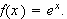The 5th Tayor polynomila for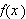is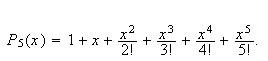and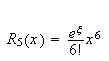Using these formulas, or otherwise, find findand for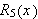for the functions

1.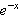2.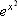2. Usefor the function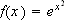to approximate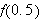and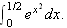Determine the accuracy of this approximation knowing that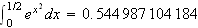.

3. Determinefor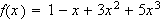and for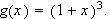4. Forfind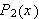and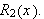Use your results to approximate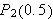and give the error exacly and using the formula for5. Findfor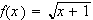and use your results to approximateandWhich result is a better approximation of the corresponding true value? Suggest a way to obtain better accuracy for the less accurate result.

6. Findforand then use your results to estimate the accuracy acheived when usingto approximateon the interval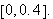7. In the previous exercise, aproximate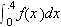andby replacingby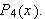8. For the function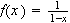find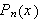and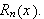Find the Taylor polynomial of least degree to approximatetoaccuracy on the interval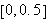and use it to approximate9. On an Excel sheet draw the graphs of the functionsand its Taylor polynomials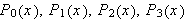over the interval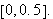This document created by Scientific WorkPlace 4.0.

Back